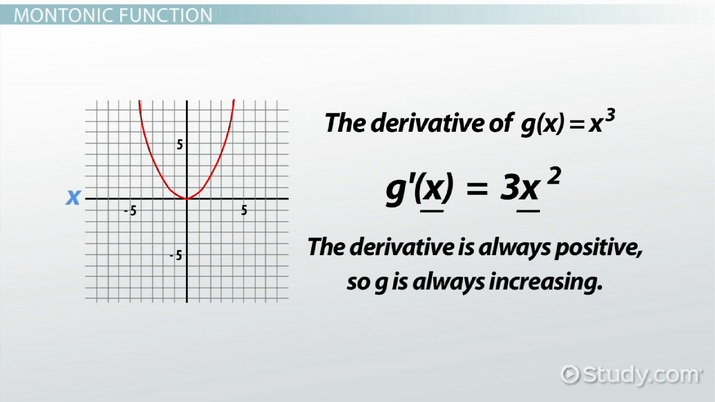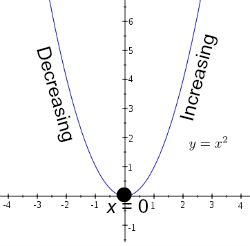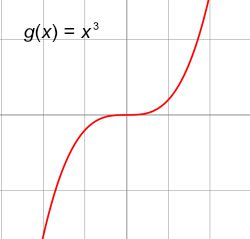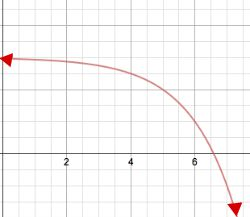# Monotonic Function: Definition & Examples

Lesson Transcript
Instructor: Laura Pennington

Laura received her Master's degree in Pure Mathematics from Michigan State University, and her Bachelor's degree in Mathematics from Grand Valley State University. She has 20 years of experience teaching collegiate mathematics at various institutions.

Explore the monotonic function, a function that graphs only as monotonic. Examine the meaning of monotonicity before delving into the monotonic and non-monotonic functions and look at several examples. Updated: 10/21/2021

## Monotonicity

The monotonicity of a function tells us if the function is increasing or decreasing. A function is increasing when its graph rises from left to right. In technical terms, a function is increasing on an interval I if for any x1 and x2 in I, x1 is less than x2 implies that f(x1) is less than f(x2). Basically, this says that when x1 is less than x2, then the function evaluated at x1 is less than the function evaluated at x2.

A function is decreasing when its graph falls from left to right. Again, the technical definition says that a function is decreasing on an interval I if for any x1 and x2 in I, x1 is less than x2 implies that f(x1) is greater than f(x2). In other words, a function is decreasing on an interval I when it is the case that whenever x1 is less than x2, f(x1) is greater than f(x2).

The monotonicity of a function is directly related to the function's derivative. A function is increasing when its derivative is positive, and a function is decreasing when its derivative is negative. For example, consider the function f(x) = x 2. The derivative is f ' (x) equals 2x. Now consider where 2x is positive and negative.

2x is greater than 0.
Divide both sides by 2.
x is greater than 0.

Therefore, f is positive when x is greater than 0, so f is increasing on the interval x is greater than 0.

Similarly, consider where 2x is negative:

2x is less than 0
Divide both sides by 2
x is less than 0

Thus, f ' is negative when x is less than 0, and f is decreasing on the interval x is less than 0.An error occurred trying to load this video.

Try refreshing the page, or contact customer support.

Coming up next: What Is an Exponential Function?

### You're on a roll. Keep up the good work!

Replay
Your next lesson will play in 10 seconds
• 0:01 Monotonicity
• 2:11 Monotonic Function
• 2:57 Non-Monotonic Function
• 3:44 Examples
• 4:41 Lesson Summary
Save Save

Want to watch this again later?

Timeline
Autoplay
Autoplay
Speed Speed## Montonic Function

When a function is increasing on its entire domain or decreasing on its entire domain, we say that the function is strictly monotonic, and we call it a monotonic function. For example, consider the function g(x) equals x 3:Notice the graph of g is increasing everywhere. Therefore, this is a monotonic function. We can also observe this by looking at the derivative of g. The derivative of g(x) equals x 3 is g' (x) equals 3x 2. Notice that no matter what we plug in for x in the derivative, it gets squared, making it positive. Thus, the derivative is always positive, so g is always increasing. Therefore, g is a monotonic function.

## Non-Monotonic Function

To solidify our understanding of what a monotonic function is, let's consider what a non-monotonic function is. A non-monotonic function is a function that is increasing and decreasing on different intervals of its domain.

For example, consider our initial example f(x) equals x 2. We saw that this function is increasing on the interval x is greater than 0, and decreasing on the interval x is less than 0. Since the function is increasing and decreasing on different intervals of its domain, the function is a non-monotonic function. Basically, if a function is not increasing on its entire domain or decreasing on its entire domain, then the function is not monotonic, and we say that it is non-monotonic.

## Examples

1.) Consider the function h. Is this function monotonic?To unlock this lesson you must be a Study.com Member.

### Register to view this lesson

Are you a student or a teacher?